Skip to main content

# Research on BeiDou anti-spoofing technology based on comprehensive radio determination satellite service

## Abstract

The BeiDou system (BDS) plays a significant role in people’s lives, but its security is easily affected by spoofing attacks. The radio determination satellite service (RDSS) is a special service of BDS that provides two-way communication, positioning, and timing services independently of the traditional radio navigation satellite service (RNSS). It can additionally be combined with RNSS to provide a comprehensive RDSS (CRDSS) service. In RDSS, after receiving a signal from the master station, the user needs to send a response signal back to the master station through a satellite. Therefore, the RDSS signal is difficult to spoof. In this study, based on the security feature of RDSS signals, an anti-spoofing method based on CRDSS is proposed to detect and mitigate spoofing attacks, verifying the advantages of the BeiDou system over other satellite navigation systems.

## Introduction

With the development of the global navigation satellite system (GNSS), satellite navigation security issues have become increasingly significant. Spoofing attacks are key issue in navigation security. Because the structure of civilian navigation signal is open to the public, a spoofing device can easily generate signals that can suppress authentic signals, thus, misleading a victim receiver to track spoofing signals. On the other hand, although military signals are encrypted, a spoofing device can still spoof a military user by replaying the authentic signals and adding delays. Spoofing signals usually lead to erroneous time delay measurements, ultimately misleading the positioning and timing results of the user.

Conventional receivers usually do not consider the impact of spoofing attacks that may result in terrible consequences. Therefore, anti-spoofing is of great significance in modern navigation applications. Anti-spoofing ability can be divided into two categories: spoofing detection and spoofing mitigation. Spoofing detection detects a spoofing attack and determines whether the navigation solution is reliable. Spoofing mitigation ensures that a user can obtain a correct navigation solution under spoofing attacks.

There have been many pieces of research on anti-spoofing. Signal quality monitoring methods detect spoofing signals from correlation peak distortion . They may falsely judge multipath signals as spoofing ones because multipath signals may also distort the correlation peak. Receiver autonomous integrity monitoring (RAIM) can inspect the measurements consistency, therefore, it can exclude one or two spoofing signals, but a great number of spoofing signals will invalidate the method . Spread spectrum security code (SSSC) and navigation message authentication (NMA) can recognize spoofing signals by encrypting a civil signal. However, these methods are not practical as they require changes to the current system . Techniques with additional sensors, such as multiple antennas, power measuring equipment, and inertial navigation systems (INSs) are usually robust, but the expense increases greatly when precise sensors are added [4,5,6]. The methods mentioned above have room for improvement. To achieve better anti-spoofing performance, more features of navigation system should be explored and utilized.

Fortunately, the BeiDou system has such features. Compared with other navigation satellite systems, the BeiDou system has the radio determination satellite service (RDSS) capability as well as the radio navigation satellite service (RNSS) capability . A standard RDSS relies on an elevation library. When a reliable elevation library is unavailable, the RDSS positioning accuracy will be very poor because the geostationary (GEO) satellites carrying the RDSS payload are all distributed in the equatorial orbit . To solve this problem, Tan Shusen proposed comprehensive RDSS (CRDSS) that combines RNSS and RDSS, thus implementing RNSS and RDSS observations simultaneously . As the frequency of an RDSS signal is different from that of an RNSS signal and the RDSS service requires two-way communication between the master station and user, it is difficult for a spoofing device to falsify RDSS signals. It can be inferred that the CRDSS method is potentially effective for anti-spoofing.

This study proposes an anti-spoofing method based on CRDSS. Under the conventional receiver architecture, this method can detect spoofing attacks and verify the correctness of the positioning results. Under a multi-peak acquisition and tracking architecture, this method can group authentic and spoofing measurements and recover correct results under spoofing attacks.

In an RNSS/RDSS dual-mode receiver, the proposed anti-spoofing method can detect and mitigate spoofing attacks that aim at either civilian or military signals without the use of any additional hardware. The proposed method demonstrates the advantages of the BeiDou system in satellite navigation security and is additionally an important contribution to the application of global navigation satellite systems.

## CRDSS spoofing detection in a conventional receiver

After a conventional receiver succeeds in acquiring and tracking a signal with a certain Pseudo-Random Noise (PRN), it will no longer try to acquire a signal with the same PRN. The RNSS pseudorange measurements can be expressed as follows:

$$\rho_{i} = [(x_{u} - x_{i} )^{2} + (y_{u} - y_{i} )^{2} + (z_{u} - z_{i} )^{2} ]^{1/2} + c\delta t_{u} ,\quad i = 1, \ldots ,K$$
(1)

here $$\rho_{i}$$ is the pseudorange measurement after correcting errors such as the satellite clock error, ionospheric delay, tropospheric delay, and relativistic effect. $$\{ x_{u} ,y_{u} ,z_{u} \}$$ is the receiver coordinate. $$\delta t_{u}$$ is the receiver clock error and $$\{ x_{i} ,y_{i} ,z_{i} \}$$ is the coordinate of satellite i. K is the number of satellites in use.

RDSS service is performed in an active positioning mode. The center station transmits C-band signals to one reference GEO satellite, and then the satellite relays the signals to an RDSS user terminal. After that, the user transmits L-band signals to several GEO satellites and the satellites relays the signals back to the center station. Taking the first GEO satellite as a reference, the RDSS pseudorange measurement after error corrections can be expressed as follows [10, 11]:

\begin{aligned} l_{j} & = [(x_{u} - x_{1} )^{2} + (y_{u} - y_{1} )^{2} + (z_{u} - z_{1} )^{2} ]^{1/2} \\ & \quad + [(x_{u} - x_{j} )^{2} + (y_{u} - y_{j} )^{2} + (z_{u} - z_{j} )^{2} ]^{1/2} ,\quad j = 1, \ldots ,L \\ \end{aligned}
(2)

The first item in the equation is the ranging measurement from the reference satellite to the user and the second item is the ranging measurement from the user to the other satellite. The distances between the center station and the satellites have been calculated and removed from the equation. L is the number of satellites in use. CRDSS uses the above observation Eqs. (1) and (2) to determine the position and clock error of the receiver. The linearized observation equation is as follows:

$$\left[ {\begin{array}{*{20}c} {f_{x}^{1} (x)} & {f_{y}^{1} (y)} & {f_{z}^{1} (z)} & 1 \\ \vdots & \vdots & \vdots & \vdots \\ {f_{x}^{K} (x)} & {f_{y}^{K} (y)} & {f_{z}^{K} (z)} & 1 \\ {g_{x}^{1,1} (x)} & {g_{y}^{1,1} (y)} & {g_{z}^{1,1} (z)} & 0 \\ \vdots & \vdots & \vdots & \vdots \\ {g_{x}^{1,L} (x)} & {g_{y}^{1,L} (y)} & {g_{z}^{1,L} (z)} & 0 \\ \end{array} } \right]\left[ {\begin{array}{*{20}c} {\Delta x_{u} } \\ {\Delta y_{u} } \\ {\Delta z_{u} } \\ {c\Delta \delta t_{u} } \\ \end{array} } \right] = \left[ {\begin{array}{*{20}c} {b_{1} } \\ \vdots \\ {b_{K} } \\ {v_{1} } \\ \vdots \\ {v_{L} } \\ \end{array} } \right]$$
(3)

where

$$f_{x}^{i} = \frac{{x_{u} - x_{i} }}{{r_{i} }},\quad f_{y}^{i} = \frac{{y_{u} - y_{i} }}{{r_{i} }},\quad f_{z}^{i} = \frac{{z_{u} - z_{i} }}{{r_{i} }}$$
(4)
$$r_{i} = [(x_{u} - x_{i} )^{2} + (y_{u} - y_{i} )^{2} + (z_{u} - z_{i} )^{2} ]^{1/2}$$
(5)
$$g_{x}^{i,j} = f_{x}^{i} + f_{x}^{j} ,\quad g_{y}^{i,j} = f_{y}^{i} + f_{y}^{j} ,\quad g_{z}^{i,j} = f_{z}^{i} + f_{z}^{j}$$
(6)
$$b_{i} = \rho_{i} - r_{i} - c\delta t_{u} ,\quad v_{j} = l_{j} - r_{1} - r_{j}$$
(7)

Equation (3) can be rewritten in the following compact form:

$$G\vec{p} = \vec{s}$$
(8)

where

$$G{ = }\left[ {\begin{array}{*{20}c} {f_{x}^{1} (x)} & {f_{y}^{1} (y)} & {f_{z}^{1} (z)} & 1 \\ \vdots & \vdots & \vdots & \vdots \\ {f_{x}^{K} (x)} & {f_{y}^{K} (y)} & {f_{z}^{K} (z)} & 1 \\ {g_{x}^{1,1} (x)} & {g_{y}^{1,1} (y)} & {g_{z}^{1,1} (z)} & 0 \\ \vdots & \vdots & \vdots & \vdots \\ {g_{x}^{1,L} (x)} & {g_{y}^{1,L} (y)} & {g_{z}^{1,L} (z)} & 0 \\ \end{array} } \right],\quad p = \left[ {\begin{array}{*{20}c} {\Delta x_{u} } \\ {\Delta y_{u} } \\ {\Delta z_{u} } \\ {c\Delta \delta t_{u} } \\ \end{array} } \right],\quad \vec{s} = \left[ {\begin{array}{*{20}c} {b_{1} } \\ \vdots \\ {b_{K} } \\ {v_{1} } \\ \vdots \\ {v_{L} } \\ \end{array} } \right]$$
(9)

Then, the residual squared sum error can be obtained as follows:

$$\varepsilon_{SSE} = \hat{s}^{T} \hat{s},\quad {\text{where}}\;\hat{s} = [I - G(G^{T} G)^{ - 1} G^{T} ]\vec{s}$$
(10)

As all the authentic signals cooperate with each other, all pseudorange observation residuals are small in a spoofing-free situation. Therefore, $$\varepsilon_{SSE}$$ is close to zero. However, when there are falsified measurements, because spoofing signals and at least one authentic RDSS signal are applied in the navigation solution, $$\varepsilon_{SSE}$$ will be very large, indicating that there are problems in the current measurements.

## CRDSS spoofing mitigation in a multi-peak acquisition and tracking receiver

In the existing multi-peak acquisition and tracking anti-spoofing method, the receiver records the largest and second largest acquisition results of the same satellite and allocates channels to track the two results. To avoid an abnormal carrier-to-noise ratio and power observations in the receiver, the power of the spoofing signal is usually not significantly higher than that of the authentic signal. Thus, when the code phases of the spoofing and authentic signals are separate, or the Doppler shift of a spoofing signal differs greatly from that of an authentic signal, the receiver can simultaneously acquire and track spoofing and authentic signals, and then extract corresponding pseudorange measurements .

To obtain the correct positioning results, it is necessary to group the measurements. This section discusses the measurements grouping problem under the multi-peak acquisition and tracking architecture, presents a measurements grouping algorithm based on CRDSS, and introduces a CRDSS spoofing mitigation method.

### Measurements grouping problem

Current measurements grouping methods mainly use multi-antenna or mobile antenna techniques to distinguish spoofing and authentic signals under the assumption that spoofing signals come from the same emitter . The methods require additional hardware or have stringent requirements for the antenna’s motion state. In addition, these methods will fail when spoofing signals come from multiple sources. Therefore, it is necessary to study new measurements grouping methods.

When a spoofing attack significantly changes the receiver clock result, all pseudorange measurements of spoofing signals will be larger or smaller than those of the authentic ones. For example, a spoofing attack that affects the time of the power management unit (PMU) in a smart grid will affect the receiver clock result but not the positioning result . In this case, we can group the measurements directly according to the numerical value of the pseudorange. Larger pseudorange measurements are categorized into one group and smaller pseudorange measurements are categorized into another group. However, the above methods will fail when the spoofing signals do not significantly change the receiver clock result. Figure 1 demonstrates such a situation. It is assumed that the spoofing signals do not change the receiver clock error. The distances from satellite i to the receiver and the spoofed position are rAi and rSi, respectively. It can be seen that rA1 > rS1 and rA2 < rS2. In this case, we cannot correctly group the measurements simply based on the values of the pseudorange measurements.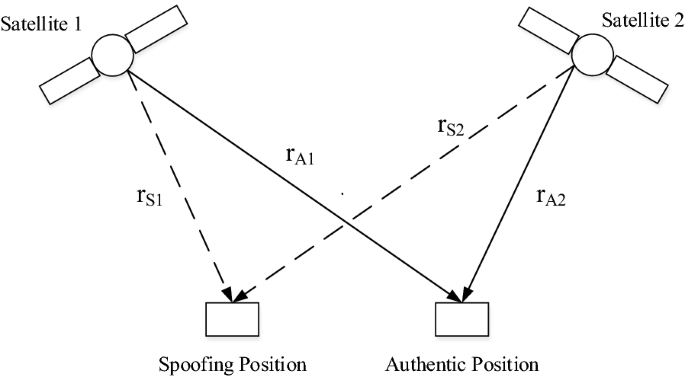Fig. 1

### Measurements grouping based on CRDSS

This study proposes a grouping algorithm based on CRDSS. The first step is to correct the clock error of the user. Assuming that the RDSS observation of the first satellite is available, according to Eqs. (1) and (2), the user clock error estimate can be calculated as follows:

$$\delta t_{u,est} = \frac{1}{2c}(2\rho_{1} - l_{1} )$$
(11)

There is a certain synergistic relationship between the pseudoranges of different authentic signals. When the receiver clock is correctly estimated, the possible user location is on a sphere whose center is the satellite position (O1) as shown in Fig. 2. When all possible positions are identified in the search range (i.e., rectangular ABCD), an arc (green line) can be obtained. As the rectangle ABCD is much smaller than the circle O2, the arc can be approximated as a straight line. The search range can be chosen as an area centered on a coarse initial location.Fig. 2

When there are multiple satellites, multiple lines can be obtained in the rectangle ABCD. Figure 3 shows simulation results using the actual ephemeris of BeiDou on the 100th day of 2018. There are spoofing and authentic signals in the simulation. The biases of the falsified position and clock are 300 m and 10 ns, respectively. The bias in z-axis is set to 0, and a search is performed in the xy plane. The results are shown in Fig. 3a. Blue lines are formed by authentic signals, and the black lines are formed by spoofing ones. It can be seen that all lines corresponding to authentic signals intersect at one point.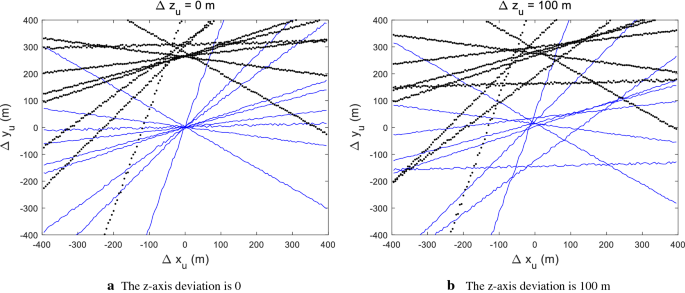Fig. 3

Figure 3b shows the results when the bias in the z-axis is 100 m. The results in this case are different from that shown in Fig. 3a, and the lines do not intersect at one point. The results show that when the receiver clock is correct, lines formed by different authentic measurements intersect at one point when the z-axis bias is 0. In other words, if we find the point of intersection in the search area, then the authentic position can be determined, and all measurements passing through the point can be categorized as authentic.

In actual situations, there are noises when we acquire measurements. Hence, the authentic signals may not pass perfectly through one point. However, since the ranging error induced by the noise signals is much smaller than that induced by the spoofing signals, the spoofing signals can still be distinguished from the authentic ones.

Based on the above phenomenon, we propose the following measurements grouping and spoofing mitigation algorithm. Figure 4 shows a diagram of the algorithm.Fig. 4
1. 1.

Select an RDSS pseudorange measurement as a reference. Without loss of generality, the number of the measurement is set to 1.

2. 2.

Sort the RNSS measurements. Assume that for the first M satellites, two different RNSS pseudorange measurements can be extracted simultaneously for each satellite, denoted as $$\rho_{k}^{(i)} ,i = 1,2;k = 1, \ldots ,M$$. For the last N satellites, only one RNSS pseudorange measurement can be extracted for each satellite, denoted as $$\rho_{k}^{(i)} ,i = 1;k = M + 1, \ldots ,M + N$$.

3. 3.

Select one RNSS observation $$\rho_{1}^{(R)}$$ corresponding to the first satellite. Here, set R = 1. Assume that this measurement is authentic. Calculate receiver clock error $$\delta t_{u,est}$$ based on Eq. (11).

4. 4.

Calculate the modified RNSS range measurements based on the user’s clock estimation:

\begin{aligned} r_{k}^{(i)} & = \rho_{k}^{(i)} - c\delta t_{u,est} ,\quad i = 1,2;k = 1, \ldots ,M \\ r_{k}^{(i)} & = \rho_{k}^{(i)} - c\delta t_{u,est} ,\quad i = 1;k = M + 1, \ldots ,M + N \\ \end{aligned}
(12)
5. 5.

Select the measurements belonging to the first M satellites: $$r_{k}^{(i)}$$, $$i = 1,2;k = 1, \ldots ,M$$.

6. 6.

Calculate statistic $$d_{min}$$ with $$r_{k}^{(i)}$$ chosen in step 5 according to the following process:

• Select possible z coordinate $$z_{u}$$ in the preset range, numbered $$i_{z}$$.

• Select possible x coordinate $$x_{u}$$ in the preset range, numbered $$i_{x}$$.

• Calculate y coordinate using the following equation:

$$y_{u,k}^{(i)} = y_{k} - [r_{k}^{(i)} - (x_{k} - x_{u} )^{2} - (z_{k} - z_{u} )^{2} ]^{1/2}$$
(13)
• Then 2M points can be obtained: $$(x_{u} [i_{x} ],y_{u,k}^{(i)} [i_{x} ,i_{z} ]),i = 1,2;k = 1, \ldots ,M$$.

• Denote $$(x_{u} [i_{x} ],y_{u,1}^{(R)} [i_{x} ,i_{z} ])$$ as point A.

• Calculate the distance from A to $$(x_{u} [i_{x} ],y_{u,k}^{(1)} [i_{x} ,i_{z} ])$$ and $$(x_{u} [i_{x} ],y_{u,k}^{(2)} [i_{x} ,i_{z} ])$$, $$k = 2, \ldots ,M$$, denoted as $$d_{A,k}^{(1)}$$ and $$d_{A,k}^{(2)}$$, respectively. Then we can obtain $$d_{A,k}^{\text{min} } = \hbox{min} \{ d_{A,k}^{(1)} ,d_{A,k}^{(2)} \}$$.

• Calculate $$d_{\text{min} }^{(1)} [i_{x} ,i_{z} ] = \sum\nolimits_{k = 2}^{M} {d_{A,k}^{\text{min} } }$$, which is the cost function corresponding to the RNSS measurement $$\rho_{1}^{(1)}$$.

7. 7.

Similarly, assume that the second RNSS measurement of satellite 1 is authentic. Set R = 2 in step 3 and repeat step 3 to 6, $$d_{\text{min} }^{(2)} [i_{x} ,i_{z} ]$$ can be obtained.

8. 8.

Calculate the final cost function: $$d_{min} [i_{x} ,i_{z} ] = \hbox{min} \{ d_{\text{min} }^{(1)} [i_{x} ,i_{z} ],d_{\text{min} }^{(2)} [i_{x} ,i_{z} ]\}$$.

9. 9.

Find the minimum value of the cost function, and get the corresponding $$x_{u}$$ and $$z_{u}$$. Then $$y_{u}$$ can be calculated with Eq. (13). $$u_{est}^{c} = [x_{u} ,y_{u} ,z_{u} ]$$ is rough estimate of the receiver position. The M measurements that are closer to $$u_{est}^{c}$$ are authentic. The remaining M measurements are falsified.

10. 10.

Deal with the left N measurements. Calculate $$r_{k}^{(i)} = \rho_{k}^{(i)} - c\delta t_{u,est}$$, $$k = M + 1, \ldots ,M + N$$ with Eq. (12), and then calculate the following statistic:

$$b_{k} = ||r_{k}^{(i)} - ||u_{k} - u_{\text{est}}^{c} ||\;||$$
(14)

where uk is the kth satellite position. If $$b_{k}$$ is smaller than a preset threshold, then $$r_{k}^{(i)}$$ and the corresponding RNSS measurement are judged as authentic.

11. 11.

Calculate the positioning results by using authentic measurements obtained in step 9 and 10. Spoofed positioning results can also be obtained using the remaining observations.

It should be noted that when measurement noise is considered, the lines of the authentic signals may not intersect exactly at one point, as shown in Fig. 3a. When the bias induced by spoofing signals is small, these signals may be mistakenly categorized as authentic ones. However, such spoofing signals will not significantly change the navigation solution, and spoofing signals which induce large bias can still be recognized and excluded.

## Simulation validation

This section provides simulation results using the CRDSS method under the conventional receiver architecture and multi-peak acquisition and tracking architecture.

### Conventional receiver architecture

In a conventional receiver architecture, only one channel is assigned to a certain PRN satellite. This section simulates two scenarios. There is no spoofing signal in the first scenario and the receiver is spoofed in the second scenario. The user’s coordinate is (40° N, 116° E, 100 m). The deception target position starts from the authentic coordinate, moves along the x-axis of the earth centred earth fixed (ECEF) coordinate at a speed of 1 m/s, and finally deviates 500 m from the authentic position. All range measurements are contaminated by Gaussian noise with zero mean and a standard deviation of 2 m. The results of $$\varepsilon_{SSE}$$ obtained with different measurements are shown in Fig. 5.Fig. 5

N in the figure is the number of RDSS measurements used in the CRDSS method. The line whose label is “spoof free” shows $$\varepsilon_{SSE}$$ when there is no spoofing signal. $$\varepsilon_{SSE}$$ is very small, demonstrating that the positioning result is reliable. Lines whose labels are “spoofed” show $$\varepsilon_{SSE}$$ when the receiver is spoofed. When no RDSS measurement is used (N = 0), $$\varepsilon_{SSE}$$ is still very small. This situation corresponds to a traditional receiver that uses only RNSS measurements. When N is not zero, $$\varepsilon_{SSE}$$ increases when the distance between spoofing position and authentic position is larger. $$\varepsilon_{SSE}$$ is slightly larger when more RDSS measurements are applied, however, the increment is very small. This demonstrates that more RDSS measurements can improve the spoofing detection performance, but the improvement is negligible.

### Multi-peak acquisition and tracking architecture

When the receiver uses a multi-peak acquisition and tracking architecture, it is possible to extract both authentic and spoofing pseudorange measurements. In this subsection, the CRDSS technique is used to group these measurements. The simulation scenario is as follows. The authentic position of the receiver is (40° N, 116° E, 100 m). The additional receiver clock bias induced by spoofing signals is 50 ns. The spoofing position is a circle centered on the authentic position with a radius of 300 m in the xy plane of the ECEF coordinate system.

Figure 6 shows the cost functions. The value of a cost function shows how well the lines in Fig. 3 intersect. The smaller the cost function, the closer the intersections of the lines. Figure 6a shows the cost function calculated with authentic RNSS measurements in the third step of the algorithm. Since the correct user clock can be estimated, the cost function is close to zero at the correct user location, corresponding to the intersection of the blue lines in Fig. 3a. Figure 6b shows the cost function calculated with the falsified RNSS measurement in the third step of the algorithm. Since the correct RDSS measurement and the falsified RNSS measurements are not consistent but are used simultaneously, the minimum value of the cost function is very large in the search area.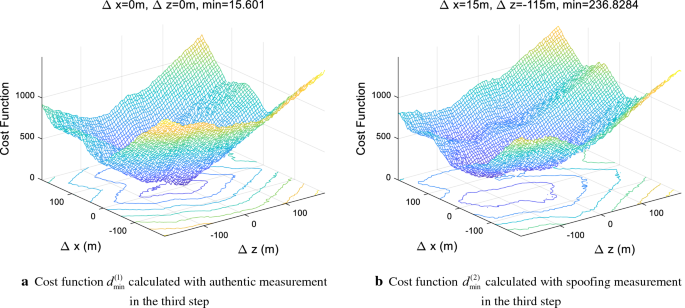Fig. 6

Figure 7a shows the minimum value of the cost functions $$d_{\text{min} }^{(1)} [i_{x} ,i_{z} ]$$ and $$d_{\text{min} }^{(2)} [i_{x} ,i_{z} ]$$ using the CRDSS method. Figure 7b shows the corresponding positioning error. The blue and red dots indicate the results when using an authentic and falsified pseudorange, respectively in Eq. (11) in the third step of the algorithm. When an authentic measurement is used in Eq. (11), the minimum values of the cost function are very small all the time, and the corresponding positioning error is close to zero. When a spoofing measurement is used in Eq. (11), the minimum values of the cost functions are large most of the time. However, at time instances of 19 and 122 s, the receiver clocks calculated with the RDSS measurement of the first satellite and the corresponding spoofing RNSS measurement turn out to be correct. Consequently, the minimum values of the cost function are very small as well and correct positioning results can be found at these epochs.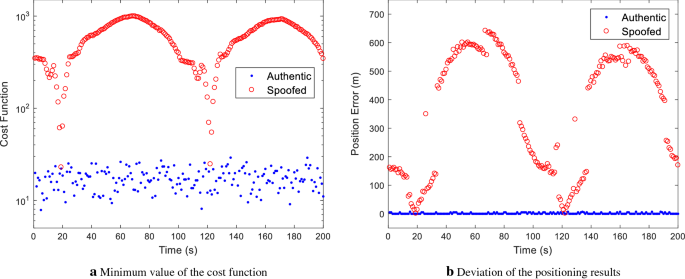Fig. 7

Figure 8 shows the positioning results obtained with different grouping methods. The black and blue dots are authentic and spoofing positioning results obtained with the CRDSS-based technique, respectively. It can be seen that the grouping method recovers the authentic positioning result. In other words, successful spoofing mitigation is implemented. The red and green dots are positioning results obtained by grouping larger and smaller pseudoranges measurements into different groups. In the simulation, the clock bias induced by the spoofing signals is only 50 ns, which is very small compared to the falsified pseudorange error. Therefore, whether a spoofing pseudorange is larger than an authentic one cannot be determined and this measurements grouping method fails. Figure 8 verifies the spoofing mitigation capability of the CRDSS-based anti-spoofing method under the multi-peak acquisition and tracking architecture.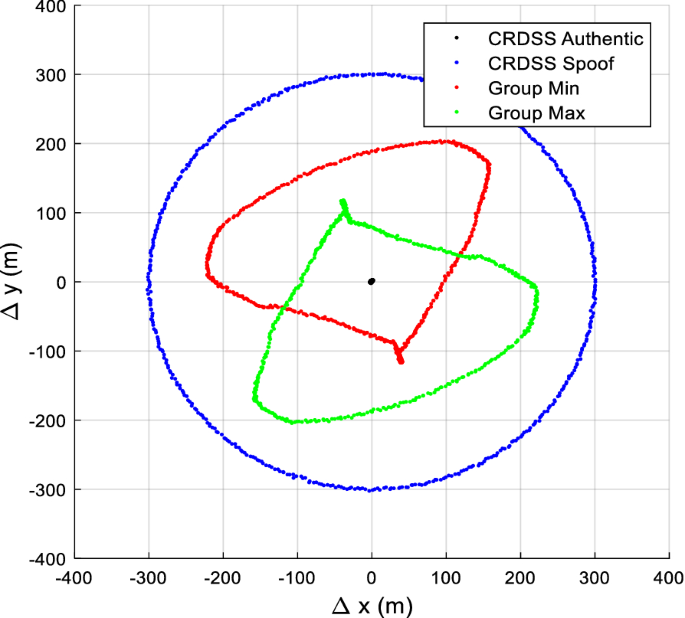Fig. 8

## Conclusions

This study proposed an anti-spoofing method based on the CRDSS. The method utilizes the security feature of the BeiDou RDSS signals. Under a conventional receiver architecture, the method can detect spoofing attacks even though all RNSS channels are taken up by spoofing signals. Under a multi-peak acquisition and tracking architecture, the method can group authentic and spoofing measurements and recover the correct positioning result. Compared with current spoofing/authentic measurements grouping techniques, the proposed method does not require additional hardware and can distinguish spoofing and authentic measurements with only a small increase in computational complexity. Thus, a good spoofing detection and a spoofing mitigation ability can be achieved for both civilian and military signals. The results show that the BeiDou system is superior to other navigation satellite systems in the area of navigation security.

## Availability of data and materials

The datasets generated during and/or analyzed during the current study are available from the corresponding author on reasonable request.

## References

1. 1.

Chao, S., Cheong, J. W., Dempster, A. G., et al. (2018). GNSS spoofing detection by means of signal quality monitoring (SQM) Metric Combinations. IEEE Access, 6, 66428–66441.

2. 2.

Shuai, H., Desi, L., Weixiao, M., et al. (2016). Antispoofing RAIM for dual-recursion particle filter of GNSS calculation. IEEE Transactions on Aerospace and Electronic Systems, 52(2), 836–851.

3. 3.

Kerns, A. J., Wesson, K. D., & Humphreys, T. E. (2014). A blueprint for civil GPS navigation message authentication. In Proceedings of the IEEE/ION PLANS meeting, Monterey, CA, USA, 5–8 May (pp. 262–269).

4. 4.

Fei, W., Hong, L., & Mingquan, L. (2018). GNSS spoofing detection based on unsynchronized double-antenna measurements. IEEE Access, 6, 31203–31212.

5. 5.

Jafarnia-Jahromi, A., Broumandan, A., Nielsen, J., et al. (2012). GPS spoofer countermeasure effectiveness based on signal strength, noise power, and C/N0 measurements. International Journal of Satellite Communications and Networking, 30, 181–191.

6. 6.

Rui, X., Mengyu, D., Ya, Q., et al. (2018). Performance analysis of GNSS/INS loosely coupled integration systems under spoofing attacks. Sensors, 18(12), 4108.

7. 7.

Jidong, C., Ranran, S., Weijie, S., & Xin, S. (2013). Positioning error research and analysis based on comprehensive RDSS method. In China Satellite Navigation Conference (CSNC) 2013 proceedings. Springer, Berlin.

8. 8.

Yulei, Y., Jing, T., & Xinyang, S. (2017). Analysis of the influence of elevation error on Beidou RDSS positioning accuracy. In Proceedings of the 6th international conference on information engineering. ACM.

9. 9.

Shusen, T. (2009). Theory and application of comprehensive RDSS position and report. Acta Geodaetica Cartographica Sinica, 38(1), 1–5.

10. 10.

Nan, X., Ranran, S., Jianhua, Z., et al. (2013). Analysis of RDSS positioning accuracy based on RNSS wide area differential technique. Science China Physics, Mechanics and Astronomy, 56(10), 1995–2001.

11. 11.

Rui, G., Ranran, S., Guangming, H., & Zhiqiao, C. (2014). Compass RDSS positioning accuracy analysis. In China Satellite Navigation Conference (CSNC) 2014 proceedings. Springer, Berlin.

12. 12.

Huiqi, T., Hong, L., & Mingquan, L. (2015) A GNSS anti-spoofing method based on the cooperation of multiple techniques. In China Satellite Navigation Conference (CSNC) 2015 proceedings (pp. 205–215). Springer, Berlin.

13. 13.

Broumandan, A., Jafarnia-Jahromi, A., & Lachapelle, G. (2015). Spoofing detection, classification and cancelation (SDCC) receiver architecture for a moving GNSS receiver. GPS Solutions, 19(3), 475–487.

14. 14.

Fan, Y., Zhang, Z., Trinkle, M., et al. (2015). A cross-layer defense mechanism against GPS spoofing attacks on PMUs in smart grids. IEEE Transactions on Smart Grid, 6(6), 2659–2668.

Download references

## Acknowledgements

The authors would thank to the reviewers for their pertinent comments.

Not applicable.

## Author information

Authors

### Contributions

All authors contribute equally to the manuscript.

### Corresponding author

Correspondence to Fei Wang.

## Ethics declarations

### Competing Interests

The authors declare that they have no competing interests.

## Additional information

### Publisher’s Note

Springer Nature remains neutral with regard to jurisdictional claims in published maps and institutional affiliations.

## Rights and permissions

Open Access This article is licensed under a Creative Commons Attribution 4.0 International License, which permits use, sharing, adaptation, distribution and reproduction in any medium or format, as long as you give appropriate credit to the original author(s) and the source, provide a link to the Creative Commons licence, and indicate if changes were made. The images or other third party material in this article are included in the article's Creative Commons licence, unless indicated otherwise in a credit line to the material. If material is not included in the article's Creative Commons licence and your intended use is not permitted by statutory regulation or exceeds the permitted use, you will need to obtain permission directly from the copyright holder. To view a copy of this licence, visit http://creativecommons.org/licenses/by/4.0/.

Reprints and Permissions